# 基于贝叶斯框架的深部成矿构造推断及不确定性研究Deep Metallogenic Structure Inference and Uncertainty Study Based on Bayesian Framework

DOI: 10.12677/AG.2020.103019, PDF, HTML, XML, 下载: 251  浏览: 1,519

Abstract: Mineral resources evaluation is now gradually moving towards 3D quantitative prediction. However, with the increase of the predicted depth, the uncertainty of the metallogenic structure located in the deep due to its scarce data has always existed. Therefore, how to reduce the uncertainty and how to measure uncertainty is a hot issue to study the 3D quantitative prediction. This paper mainly uses the Bayesian theory to construct the Bayesian model, and based on this model, the Bayesian inference is made for the deep metallogenic structure of the Dayingezhuang mining area, and finally obtains a visual model based on the deep metallogenic structure. Research shows that the uncertainty visualization model obtained through this example is consistent with geological research, and can provide guidance for subsequent iterative inference of 3D models of deep metallogenic structures.

1. 引言

2. 研究区地质概况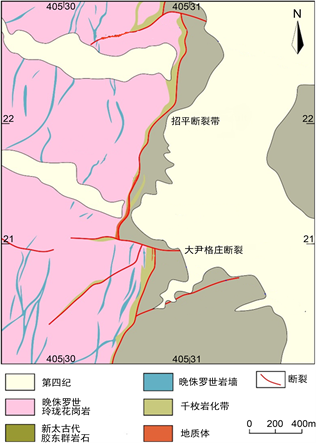Figure 1. Plan of Zhaoping fault (revisioned from Mao, X. et al., 2019 )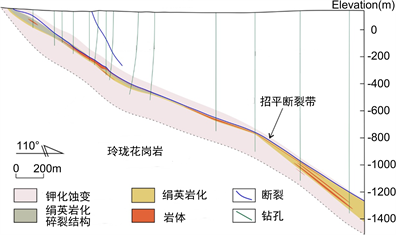Figure 2. Section of Zhaoping fault (revisioned from Mao, X. et al., 2019 )

3. 方法

3.1. 数据介绍

3.1.1. 初始三维地质线串模型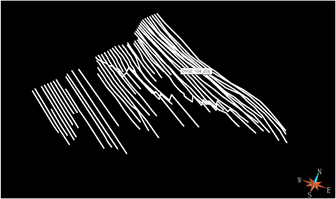Figure 3. Dayingezhuang fault string model

3.1.2. 重力异常数据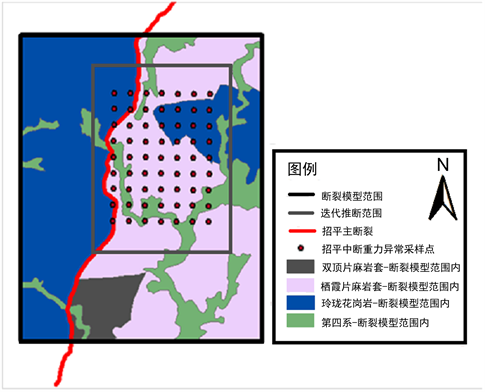Figure 4. Distribution of sampling points for ground gravity anomalies in Dayingezhuang

3.1.3. 岩性密度数据Table 1. System resulting data of standard experiment (unit: g/cm3)Table 2. Samples of physical parameters of hanging wall and foot wall (unit: g/cm3)

3.2. 贝叶斯模型

$P\left(A|B\right)=\frac{P\left(B|A\right)P\left(A\right)}{P\left(B\right)}$ (1)

$P\left(F|\mathcal{E}\right)=\frac{p\left(F\right)P\left(\mathcal{E}|F\right)}{P\left(\mathcal{E}\right)}$ (2)

$P\left(F|\mathcal{E}\right)\propto p\left(F\right)P\left(\mathcal{E}|F\right)$(3)

3.2.1. 似然项

$P\left(\mathcal{E}|F\right)={\int }_{\theta }P\left(\mathcal{E}|F,\theta \right)P\left(\theta \right)\text{d}\theta$ (4)

1) 核密度估计：核密度估计属于一种非参数估计方法，该方法不需要给定数据的先验分布，它是通过数据样本本身出发来估计数据的分布特征 。我们通过收集到上下盘岩性的密度样本，利用核密度估计得到岩性密度的概率分布。

$P\left(\theta \right)={f}_{h}\left(\theta \right)=\frac{1}{nh}\underset{i=1}{\overset{n}{\sum }}K\left(\frac{\theta -{\theta }_{i}}{h}\right)$ (5)

2) 重力异常正演：我们选取了位于大尹格庄地表63个观测点的布格重力异常值，假设地面每个观测

${G}_{i}$ 服从独立同分布，因此 $P\left(\mathcal{E}|F,\theta \right)$ 可写成如 ${\prod }_{i=1}^{n}P\left({\mathcal{E}}_{i}|F,\theta \right)$ 的连乘形式。每项 $P\left({\mathcal{E}}_{i}|F,\theta \right)$ 可表示为：

$P\left({\mathcal{E}}_{i}|F,\theta \right)=\frac{1}{\sqrt{2\pi }\sigma }\mathrm{exp}\left(-\frac{{\left({\mathcal{E}}_{i}-fw{d}_{F,\theta }\right)}^{2}}{2{\sigma }^{2}}\right)$ (6)

$fw{d}_{F,\theta }$ 表示地表观测点的正演计算值。计算公式  为：(7)(8)

${r}_{ijk}=\sqrt{{x}_{i}^{2}+{y}_{j}^{2}+{z}_{k}^{2}}$ (9)

${\mu }_{ijk}={\left(-1\right)}^{i}{\left(-1\right)}^{j}{\left(-1\right)}^{k}$ (10)

3.2.2. 先验项(11)

$E\left(F\right)=\lambda \left(z\right){‖F-{F}_{0}‖}^{2}$ (12)

3.3. 贝叶斯推断

MCMC采样方法会根据给定的一个初始值或者一个初始向量，可以采样出下一符合要求的样本，且当前样本只与前一次样本有关。通过每次采出的样本，设置接受率，再根据给定的接受率来判断当前样本是否被接受，如果是，则作为下一次采样的起始样本。MCMC接受率  表示如式(13)所示：

$\mathrm{min}\left(1,\frac{\pi \left({z}^{*}\right)q\left(z|{z}^{*}\right)}{\pi \left(z\right)q\left({z}^{*}|z\right)}\right)$ (13)

$P\left(\mathcal{E}|F\right)={\int }_{\theta }P\left(\mathcal{E}|F,\theta \right)P\left(\theta \right)\text{d}\theta =\frac{1}{n}{\sum }^{\text{​}}P\left(\mathcal{E}|F,{\theta }_{i}\right)P\left({\theta }_{i}\right)$ (14)

3.4. 信息熵

$H\left(x\right)=-\underset{i=1}{\overset{n}{\sum }}\text{ }p\left({x}_{i}\right)\mathrm{log}\left(p\left({x}_{i}\right)\right)$ (15)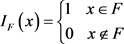(16)

${P}_{F}\left(x\right)={\sum }^{\text{​}}\frac{{I}_{F}\left(x\right)}{n}$ (17)

4. 结果

4.1. 物性参数分布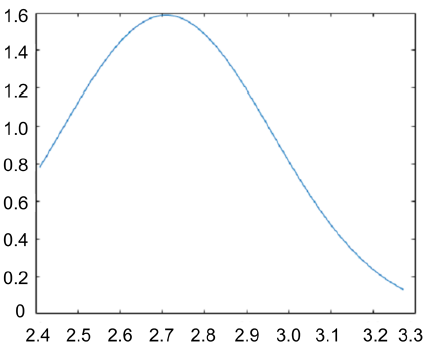(a)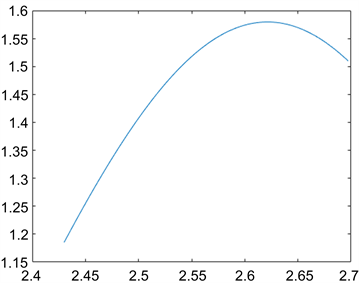(b)

Figure 5. (a) Physical Property Probability Density Distribution of hanging wall; (b) Physical Property Probability Density Distribution of foot wall

4.2. 不确定性可视化

4.2.1 . 物性参数样本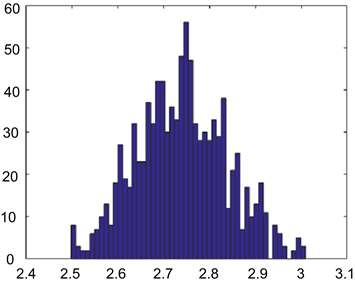(a)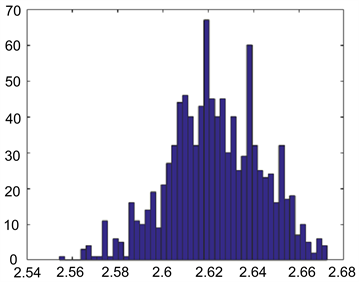(b)

Figure 6. (a) Physical parameters Samples of hanging wall; (b) physical parameters Samples of foot wall

4.2.2 . 信息熵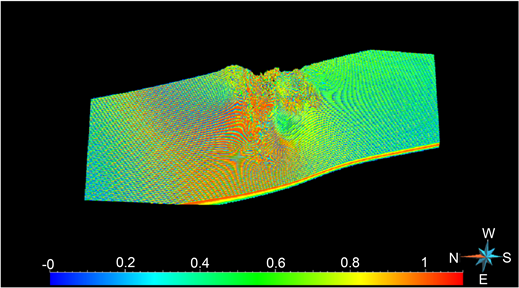Figure 7. Three-dimensional model information entropy of deep metallogenic structure

5. 结论

1) 本文根据大尹格庄矿床钻孔的物性参数采样样本，利用核密度估计方法得到了位于该研究区域断裂的上下盘密度概率分布，为地质三维模型不确定性研究提供非常重要的基础。

2) 本文根据大尹格庄矿床重力异常数据，建立贝叶斯框架中的似然函数，并结合MCMC方法推断了招平断裂面形态的后验概率分布，基于此后验概率，采用信息熵方法对三维地质模型的不确定性进行了可视化。通过结果可发现出地下地质特征的复杂性和数据的有限性是造成模型不确定性的主要原因。通过计算得到的可视化模型是建立在当前输入的三维地质模型基础上，这可以很直观的展现该模型的不确定性，有助于引导三维地质模型修正工作沿着不确定性更小的部位开展深部三维结构重建。

  Zhao, P. (1992) Theories, Principles, and Methods for the Statistical Prediction of Mineral Deposits. Mathematical Geology, 24, 589-595. https://doi.org/10.1007/BF00894226  肖克炎. 大比例尺综合信息成矿预测的研究问题及途径[J]. 黄金地质科技, 1993(4): 34-39.  Caumon, G., Ortiz, J.M. and Rabeau, O. (2006) A Comparative Study of Three Da-ta-Driven Mineral Potential Mapping Techniques. In: IAMG06, Belgium.  成秋明. 成矿过程奇异性与矿产预测定量化的新理论与新方法[J]. 地学前缘, 2007, 14(5): 42-53.  Wang, G., Zhang, S., Yan, C., Song, Y., Sun, Y., Li, D. and Xu, F. (2011) Mineral Potential Targeting and Resource Assessment Based on 3D Geological Modeling in Luanchuan Region, China. Computers & Geosciences, 37, 1976-1988. https://doi.org/10.1016/j.cageo.2011.05.007  陈建平, 吕鹏, 吴文, 等. 基于三维可视化技术的隐伏矿体预测[J]. 地学前缘, 2007, 14(5): 54-62.  毛先成. 三维数字矿床与隐伏矿体立体定量预测研究[D]: [博士学位论文]. 长沙: 中南大学, 2006: 1-100.  袁峰, 李晓晖, 张明明. 隐伏矿体三维综合信息成矿预测方法[J]. 地质学报, 2014, 88(4): 630-643.  Martin, L., Perron, G. and Masson, M. (2007) Discovery from 3D Visualization and Quantitative Modelling. In: Proceedings of Exploration 07: Fifth DecenNial Interna-tional Conference on Mineral Exploration, Toronto.  赵鹏大. 成矿定量预测与深部找矿[J]. 地学前缘, 2007, 14(5): 1-10.  滕吉文, 张永谦, 阮小敏, 等. 地球内部壳幔介质地震各向异性与动力学响应[J]. 地球物理学报, 2012, 55(11): 3648-3670.  吕庆田, 刘振东, 严加永, 等. 铜陵矿集区地壳结构格架: 反射地震证据[C]//2014年中国地球科学联合学术年会, 2014年10月20日~23日, 北京.  曾广兵, 高星, 高凤. 招平断裂带大尹格庄–夏甸段控矿构造性质分析[C]//2016年第一届今日财富论坛论文集. 北京: 今日财富杂志社, 2016: P618.51.  高星, 杨斌, 陈艳, 等. 山东大尹格庄金矿床控矿构造性质与演化[J]. 矿床地质, 2010, 29(S1): 45-46.  Mao, X., Ren, J., Liu, Z., et al. (2019) Three-Dimensional Prospectivity Modeling of the Jiaojia-Type Gold Deposit, Jiaodong Peninsula, Eastern China: A Case Study of the Dayingezhuang Deposit. Journal of Geochemical Exploration, 203, 27-44. https://doi.org/10.1016/j.gexplo.2019.04.002  Pearl, J. (1986) Fusion, Propagation, and Structuring in Belief Net-works. Artificial Intelligence, 29, 241- 288. https://doi.org/10.1016/0004-3702(86)90072-X  肖张波. 地震数据约束下的贝叶斯随机反演方法研究[D]: [硕士学位论文]. 青岛: 中国石油大学(华东), 2013.  李东利, 孟强, 赵明. 边坡稳定不确定性分析的贝叶斯方法[J]. 河南城建学院学报, 2013, 22(5): 9-13.  胥新政, 强毅, 傅华栋. 基于贝叶斯方法的不确定性信息处理研究进展综述[J]. 机电产品开发与创新, 2018, 31(6): 1-2+9.  曹哲铭, 尹立子. 浅析核密度估计方法[J]. 中国科技博览, 2014(37): 345.  李存华, 孙志挥, 陈耿, 等. 核密度估计及其在聚类算法构造中的应用[J]. 计算机研究与发展, 2004, 41(10): 1712-1719.  Bhattacharyya, B.K. (1964) Magnetic Anomalies Due to Prism-Shaped Bodies with Arbi-trary Polarization. Geophysics, 29, 517-531. https://doi.org/10.1190/1.1439386  王建平, 程声通, 贾海峰. 基于MCMC法的水质模型参数不确定性研究[J]. 环境科学, 2006, 27(1): 24-32.  房爱东, 谢士春. MCMC采样技术及其在贝叶斯推断上的应用[J]. 长沙大学学报, 33(2): 6-10.  Núñez, J.A., Cincotta, P.M. and Wachlin, F.C. (1996) In-formation Entropy. Celestial Mechanics & Dynamical Astronomy, 64, 43-53. https://doi.org/10.1007/BF00051604